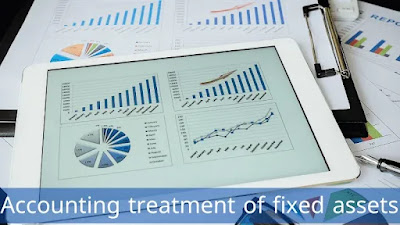# Accounting treatment of fixed assets

### Fixed assets

The concept of fixed assets is called those assets that are acquired by the organization for the purposes of use in the conduct of the business of the organization and help it to carry out the activities that are specialized to it, and does not include in the concept of fixed assets assets that are acquired for the purposes of sale or investment, and the most prominent characteristic of this type of assets is the length The relative duration of the acquisition, which is often five years or more, and the accounting treatment of fixed assets is of great importance to the organization because of their relatively high material value, and in this article the accounting treatment of fixed assets will be clarified.

### Accounting treatment of fixed assets

The accounting treatment of fixed assets comes from the nature of fixed assets for the facility, as it does not retain its book value in which it is purchased over time, but this value gradually decreases, and the accounting treatment of fixed assets is done through the presence of a depreciation account in which the depreciation expense is included to reduce the value of the assets With the passage of years, the depreciation expense account on fixed assets is included in the balance sheet, in addition, once the fixed asset is acquired, its purchase process reduces the organization's cash account by the amount of the asset's book value recorded at the moment of purchase.Accounting treatment of fixed assets

There is what is known as the useful life of a fixed asset, which consists of a specific number of years in which the book value of the fixed asset is depreciated over time at the end of the fiscal year, so that the accounting treatment of the depreciation expense of the fixed asset increases the value of the cumulative expense of the fixed asset in each year to equal the cumulative expense value The historical carrying amount of the fixed asset at the end of the useful life, and at the same time, the value of the fixed asset at the end of the useful life is zero due to its annual decrease and reaching the value of zero in the last year of the useful life.

### Fixed Assets Financial Ratio

In addition to the accounting treatment of fixed assets, there is a set of financial ratios and equations through which the interest realized from the acquisition of fixed assets is evaluated, and the impact of the acquisition of the fixed asset and the return it caused as a result of the organization’s acquisition of it, and there is a set of financial ratios and equations that clarify Among the most prominent of that are the following:

#### Fixed Asset Turnover

The fixed asset turnover rate is one of the most important financial ratios that reflects the organization’s ability to achieve sales from its normal activity due to the presence of fixed assets that have been acquired by the organization. Effectiveness in the production of goods to be sold, and the fixed asset turnover rate is calculated through the following equation:
Fixed Asset Turnover Ratio = Net Sales/Net Fixed Assets

#### return on assets

The return on assets ratio is one of the profitability ratios through which the efficiency of using fixed assets in generating profit is evaluated as a percentage. The ratio that is calculated expresses the amount of profit generated from investing in assets, and this ratio is calculated through the following equation:
Return on Assets = Net Income / Total Assets